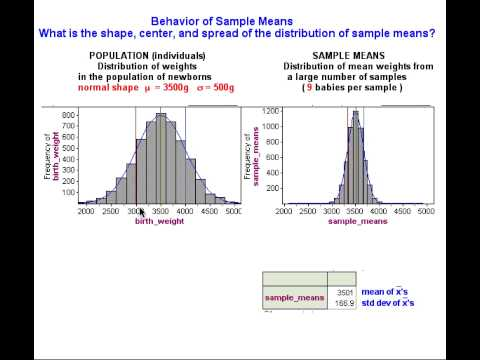# 10.13: Distribution of Sample Means (1 of 4)

$$\newcommand{\vecs}{\overset { \rightharpoonup} {\mathbf{#1}} }$$ $$\newcommand{\vecd}{\overset{-\!-\!\rightharpoonup}{\vphantom{a}\smash {#1}}}$$$$\newcommand{\id}{\mathrm{id}}$$ $$\newcommand{\Span}{\mathrm{span}}$$ $$\newcommand{\kernel}{\mathrm{null}\,}$$ $$\newcommand{\range}{\mathrm{range}\,}$$ $$\newcommand{\RealPart}{\mathrm{Re}}$$ $$\newcommand{\ImaginaryPart}{\mathrm{Im}}$$ $$\newcommand{\Argument}{\mathrm{Arg}}$$ $$\newcommand{\norm}{\| #1 \|}$$ $$\newcommand{\inner}{\langle #1, #2 \rangle}$$ $$\newcommand{\Span}{\mathrm{span}}$$ $$\newcommand{\id}{\mathrm{id}}$$ $$\newcommand{\Span}{\mathrm{span}}$$ $$\newcommand{\kernel}{\mathrm{null}\,}$$ $$\newcommand{\range}{\mathrm{range}\,}$$ $$\newcommand{\RealPart}{\mathrm{Re}}$$ $$\newcommand{\ImaginaryPart}{\mathrm{Im}}$$ $$\newcommand{\Argument}{\mathrm{Arg}}$$ $$\newcommand{\norm}{\| #1 \|}$$ $$\newcommand{\inner}{\langle #1, #2 \rangle}$$ $$\newcommand{\Span}{\mathrm{span}}$$$$\newcommand{\AA}{\unicode[.8,0]{x212B}}$$

In the next example, we predict what happens in the long run when we select many, many random samples of 9 babies at a time from a population with a mean birth weight of 3,500 grams. Then we watch a simulation to see if our predictions are correct.

Example

## Predicting the Behavior of Mean Birth Weights

Note: Means of samples randomly selected from a population are consequently random variables themselves because the means of random samples vary unpredictably in the short run but have a predictable pattern in the long run. Based on our intuition, what we experienced with the simulation, and what we learned about the behavior of samples in previous modules, we might expect the following about the distribution of sample means that come from a population where µ = 3,500:

Center: Some sample means will be on the low side – say 3,000 grams or so – while others will be on the high side – say 4,000 grams or so. In repeated sampling, we might expect that the random samples will average out to the underlying population mean of 3,500 grams. In other words, the mean of the sample means will be µ. This is exactly what we observed in the case of proportions in Linking Probability to Statistical Inference. There, the mean of sample proportions was the population proportion.

Spread: For large samples, we might expect that sample means will not stray too far from the population mean of 3,500. Sample means lower than 3,000 or higher than 4,000 might be surprising. For smaller samples, we would be less surprised by sample means that varied quite a bit from 3,500. In others words, we might expect greater variability in sample means for smaller samples. So sample size again plays a role in the spread of the distribution of sample statistics, just as we observed for sample proportions.

Shape: Sample means closest to 3,500 will be the most common, with sample means far from 3,500 in either direction progressively less likely. In other words, the shape of the distribution of sample means should be somewhat normal. This, again, is what we saw when we looked at sample proportions.

The discussion of shape, center, and spread here is not very specific. We work toward making these statements more specific over the next two pages.

Now let’s see if our predictions about the sampling distribution are correct. In the next simulation, we randomly select thousands of random samples of 9 babies each.

## WalkThrough SimulationA YouTube element has been excluded from this version of the text. You can view it online here: pb.libretexts.org/cis/?p=404

The distribution of the values of the sample mean$\overline{x}$ in repeated samples is called the sampling distribution of$\overline{X}$.

At this point, you may be wondering if we should use a larger sample to answer our question. Will our conclusion change if we increase the number of babies in the sample? We investigate this question next.